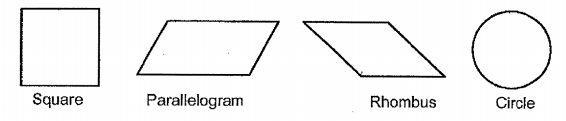# Primary 6 SA2 Practice #4

Time elapsed:
Q1. In 42.315, what does the digit 3 stand for?     [1 mark]
Q2. The figure below is made up of a rectangle and 3 identical circles. Find the area of the shaded part. Leave your answer in terms of π.     [2 marks]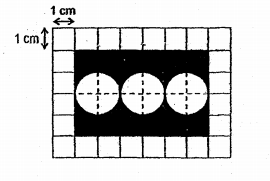Q3. At 8 a.m., a swimming pool was completely filled with water. From 8 a.m. to 1 p.m., water was drained from the swimming pool. The line graph below shows the volume of water in the swimming pool from 8 a.m. to 1 p.m. During which one-hour period was the decrease in the volume of water the greatest?     [1 mark]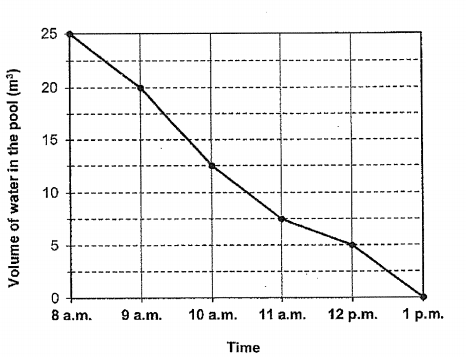Q4. Kate paid \$12 for 30 identical pencils. What is the cost of each pencil?     [1 mark]
Q5. The table below shows how Felicia spent her money. Which of the following bar graph best represents Felicia's spending?     [1 mark]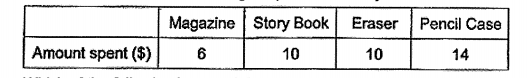Q6. The empty cuboid below measures 8 cm by 9 cm by 15 cm. Find the area of the shaded face.     [1 mark]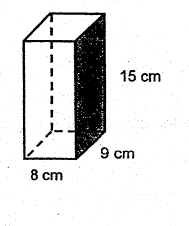Q7. Read the riddle below. Which one of the following is the correct shape?     [1 mark]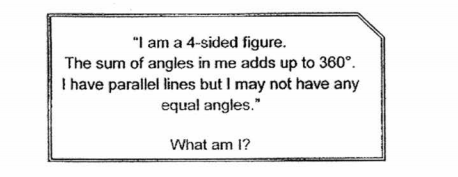Q8. Vivian bought 56 kg of minced meat. She gave 14 of it to her neighbour and 13 of the remainder to George. How much minced meat did Vivian have left?     [2 marks]
Q9. What is the missing number in the blank? 5 23 = 4 _ 3     [1 mark]
Q10. Express 56 ÷ 2 in its simplest form.     [1 mark]
Q11. Isabelle bought some sugar. She used 13 of the sugar on Monday and 25 of it on Tuesday. The amount of sugar she had left was 132 g. How much sugar did Isabelle buy?     [2 marks]
Q12. The price of a mobile phone is \$200 excluding GST. GST is 7%. What is the price of the mobile phone including GST?     [1 mark]
Q13. Natasha cut out three identical right-angled triangles. She joined them to form the figure below. AB = 10 cm and AC = 6 cm. The perimeter of the figure is 56 cm. Find the area of Triangle ABC.     [2 marks]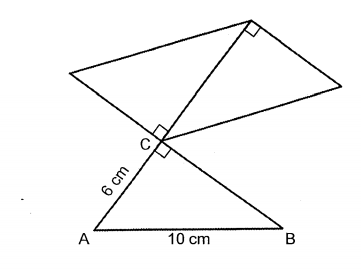Q14. There were 1600 participants at a conference. 80% of them were female. Some female participants left the conference and the ratio of the number of female participants to the number of male participants became 7 : 4. How many female participants left the conference?     [2 marks]
Q15. What is the difference between 13 tens and 13 tenths?     [2 marks]
Q16. Study the straight lines on the protractor below. Which two angles are equal?     [1 mark]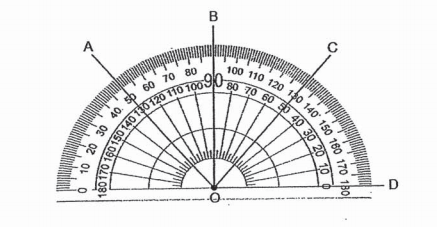Q17. Which of the following numbers is 12 000 when rounded to the nearest hundred?     [1 mark]
Q18. Find the value of (260 - 80 + 120) ÷ (10 - 4).     [1 mark]
Q19. Arrange the fractions, 53, 1 58, 119, from the smallest to the largest.     [1 mark]
Q20. How many of the following figures have at least one line of symmetry?     [1 mark]